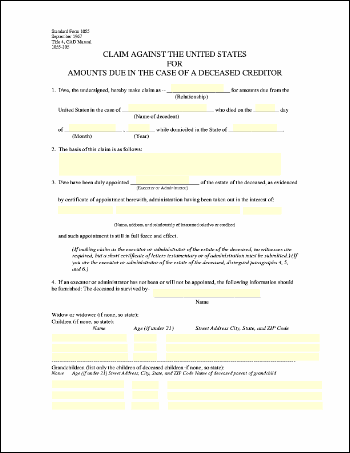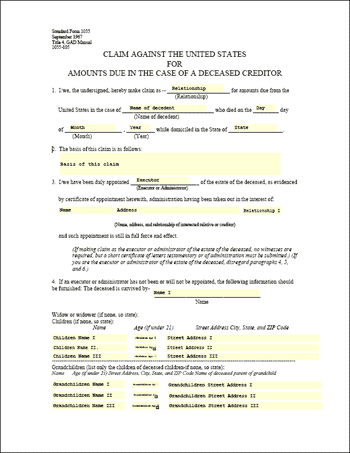eForm Fields ExampleThis example shows how to change the values of eForm fields. In this example, we simply set the contents of each field in a form with the name of that field.Src

 First, we create an ABCpdf Doc object and read in our template form. ```Set theDoc = Server.CreateObject("ABCpdf11.Doc") theDoc.Read "c:\mypdfs\form.pdf"```We iterate through each of the top-level fields. For each field, we set the value of the field to be equal to the value of the name. ```For Each theField in theDoc.Form.Fields   theField.Value = theField.Name Next```Finally, we save. `theDoc.Save "c:\mypdfs\eformfields.pdf"`Given the following document.form.pdf This is the kind of output you might expect.eformfields.pdf